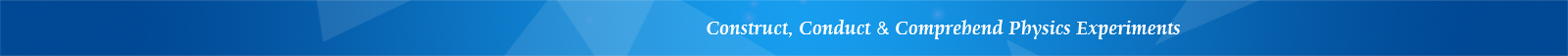## LEEI-50 Experimental Apparatus of PN Junction Characteristics

Features

• PN junction properties

• Boltzmann constant measurement

• Electronics for physics applications

Introduction

The physical properties of a PN junction are important parameters in general physics, semiconductor physics and electronics. The Boltzmann constant is one of the important fundamental constants in physics. This experimental apparatus can be used to measure the physical properties of a PN junction and the Boltzmann constant. It can also be used as a learning tool for measuring weak current. The instrument includes thermostats controller and platinum resistance thermometric bridge, and can hence measure the relationship between PN junction voltage and thermodynamic temperature, calculate the sensitivity of a temperature sensor, and obtain the prohibited bandwidth of a silicon material at 0 K approximately. This instrument is designed for teaching general physics at colleges and universities.

Using this instrument, the following experiments can be performed:

1. Measure PN junction diffusion current versus junction voltage, and acquire Boltzmann constant

2. Construct a current-voltage converter using operational amplifiers for measuring weak current

3. Measure PN junction voltage versus temperature, and acquire the sensitivity of junction voltage with temperature

4. Obtain prohibited bandwidth of silicon material at 0 K approximately

5. Measure temperature and electrical resistance using platinum resistance and DC bridge method